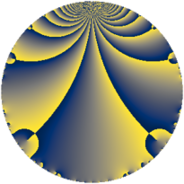# Properties

 Label 8470.2.a.byLevel $8470$ Weight $2$ Character orbit 8470.a Self dual yes Analytic conductor $67.633$ Analytic rank $1$ Dimension $2$ CM no Inner twists $1$

# Related objects

## Newspace parameters

 Level: $$N$$ $$=$$ $$8470 = 2 \cdot 5 \cdot 7 \cdot 11^{2}$$ Weight: $$k$$ $$=$$ $$2$$ Character orbit: $$[\chi]$$ $$=$$ 8470.a (trivial)

## Newform invariants

 Self dual: yes Analytic conductor: $$67.6332905120$$ Analytic rank: $$1$$ Dimension: $$2$$ Coefficient field: $$\Q(\sqrt{5})$$ Defining polynomial: $$x^{2} - x - 1$$ Coefficient ring: $$\Z[a_1, a_2, a_3]$$ Coefficient ring index: $$2$$ Twist minimal: yes Fricke sign: $$1$$ Sato-Tate group: $\mathrm{SU}(2)$

## $q$-expansion

Coefficients of the $$q$$-expansion are expressed in terms of $$\beta = \sqrt{5}$$. We also show the integral $$q$$-expansion of the trace form.

 $$f(q)$$ $$=$$ $$q + q^{2} -\beta q^{3} + q^{4} - q^{5} -\beta q^{6} - q^{7} + q^{8} + 2 q^{9} +O(q^{10})$$ $$q + q^{2} -\beta q^{3} + q^{4} - q^{5} -\beta q^{6} - q^{7} + q^{8} + 2 q^{9} - q^{10} -\beta q^{12} - q^{13} - q^{14} + \beta q^{15} + q^{16} + ( -1 + \beta ) q^{17} + 2 q^{18} + \beta q^{19} - q^{20} + \beta q^{21} + ( -6 + \beta ) q^{23} -\beta q^{24} + q^{25} - q^{26} + \beta q^{27} - q^{28} -2 \beta q^{29} + \beta q^{30} + ( -3 - \beta ) q^{31} + q^{32} + ( -1 + \beta ) q^{34} + q^{35} + 2 q^{36} + 4 \beta q^{37} + \beta q^{38} + \beta q^{39} - q^{40} + ( 5 + \beta ) q^{41} + \beta q^{42} + ( -1 - \beta ) q^{43} -2 q^{45} + ( -6 + \beta ) q^{46} + ( 1 + 3 \beta ) q^{47} -\beta q^{48} + q^{49} + q^{50} + ( -5 + \beta ) q^{51} - q^{52} + ( 1 - 3 \beta ) q^{53} + \beta q^{54} - q^{56} -5 q^{57} -2 \beta q^{58} + ( -2 + 3 \beta ) q^{59} + \beta q^{60} + ( 8 - 2 \beta ) q^{61} + ( -3 - \beta ) q^{62} -2 q^{63} + q^{64} + q^{65} + ( -5 - \beta ) q^{67} + ( -1 + \beta ) q^{68} + ( -5 + 6 \beta ) q^{69} + q^{70} + ( -6 + 2 \beta ) q^{71} + 2 q^{72} + ( 5 + \beta ) q^{73} + 4 \beta q^{74} -\beta q^{75} + \beta q^{76} + \beta q^{78} + ( -8 - \beta ) q^{79} - q^{80} -11 q^{81} + ( 5 + \beta ) q^{82} + ( -2 + \beta ) q^{83} + \beta q^{84} + ( 1 - \beta ) q^{85} + ( -1 - \beta ) q^{86} + 10 q^{87} + ( -15 - \beta ) q^{89} -2 q^{90} + q^{91} + ( -6 + \beta ) q^{92} + ( 5 + 3 \beta ) q^{93} + ( 1 + 3 \beta ) q^{94} -\beta q^{95} -\beta q^{96} + ( 8 - 2 \beta ) q^{97} + q^{98} +O(q^{100})$$ $$\operatorname{Tr}(f)(q)$$ $$=$$ $$2q + 2q^{2} + 2q^{4} - 2q^{5} - 2q^{7} + 2q^{8} + 4q^{9} + O(q^{10})$$ $$2q + 2q^{2} + 2q^{4} - 2q^{5} - 2q^{7} + 2q^{8} + 4q^{9} - 2q^{10} - 2q^{13} - 2q^{14} + 2q^{16} - 2q^{17} + 4q^{18} - 2q^{20} - 12q^{23} + 2q^{25} - 2q^{26} - 2q^{28} - 6q^{31} + 2q^{32} - 2q^{34} + 2q^{35} + 4q^{36} - 2q^{40} + 10q^{41} - 2q^{43} - 4q^{45} - 12q^{46} + 2q^{47} + 2q^{49} + 2q^{50} - 10q^{51} - 2q^{52} + 2q^{53} - 2q^{56} - 10q^{57} - 4q^{59} + 16q^{61} - 6q^{62} - 4q^{63} + 2q^{64} + 2q^{65} - 10q^{67} - 2q^{68} - 10q^{69} + 2q^{70} - 12q^{71} + 4q^{72} + 10q^{73} - 16q^{79} - 2q^{80} - 22q^{81} + 10q^{82} - 4q^{83} + 2q^{85} - 2q^{86} + 20q^{87} - 30q^{89} - 4q^{90} + 2q^{91} - 12q^{92} + 10q^{93} + 2q^{94} + 16q^{97} + 2q^{98} + O(q^{100})$$

## Embeddings

For each embedding $$\iota_m$$ of the coefficient field, the values $$\iota_m(a_n)$$ are shown below.

For more information on an embedded modular form you can click on its label.

Label $$\iota_m(\nu)$$ $$a_{2}$$ $$a_{3}$$ $$a_{4}$$ $$a_{5}$$ $$a_{6}$$ $$a_{7}$$ $$a_{8}$$ $$a_{9}$$ $$a_{10}$$
1.1
 1.61803 −0.618034
1.00000 −2.23607 1.00000 −1.00000 −2.23607 −1.00000 1.00000 2.00000 −1.00000
1.2 1.00000 2.23607 1.00000 −1.00000 2.23607 −1.00000 1.00000 2.00000 −1.00000
 $$n$$: e.g. 2-40 or 990-1000 Significant digits: Format: Complex embeddings Normalized embeddings Satake parameters Satake angles

## Atkin-Lehner signs

$$p$$ Sign
$$2$$ $$-1$$
$$5$$ $$1$$
$$7$$ $$1$$
$$11$$ $$-1$$

## Inner twists

This newform does not admit any (nontrivial) inner twists.

## Twists

By twisting character orbit
Char Parity Ord Mult Type Twist Min Dim
1.a even 1 1 trivial 8470.2.a.by yes 2
11.b odd 2 1 8470.2.a.bn 2

By twisted newform orbit
Twist Min Dim Char Parity Ord Mult Type
8470.2.a.bn 2 11.b odd 2 1
8470.2.a.by yes 2 1.a even 1 1 trivial

## Hecke kernels

This newform subspace can be constructed as the intersection of the kernels of the following linear operators acting on $$S_{2}^{\mathrm{new}}(\Gamma_0(8470))$$:

 $$T_{3}^{2} - 5$$ $$T_{13} + 1$$ $$T_{17}^{2} + 2 T_{17} - 4$$ $$T_{19}^{2} - 5$$

## Hecke characteristic polynomials

$p$ $F_p(T)$
$2$ $$( -1 + T )^{2}$$
$3$ $$-5 + T^{2}$$
$5$ $$( 1 + T )^{2}$$
$7$ $$( 1 + T )^{2}$$
$11$ $$T^{2}$$
$13$ $$( 1 + T )^{2}$$
$17$ $$-4 + 2 T + T^{2}$$
$19$ $$-5 + T^{2}$$
$23$ $$31 + 12 T + T^{2}$$
$29$ $$-20 + T^{2}$$
$31$ $$4 + 6 T + T^{2}$$
$37$ $$-80 + T^{2}$$
$41$ $$20 - 10 T + T^{2}$$
$43$ $$-4 + 2 T + T^{2}$$
$47$ $$-44 - 2 T + T^{2}$$
$53$ $$-44 - 2 T + T^{2}$$
$59$ $$-41 + 4 T + T^{2}$$
$61$ $$44 - 16 T + T^{2}$$
$67$ $$20 + 10 T + T^{2}$$
$71$ $$16 + 12 T + T^{2}$$
$73$ $$20 - 10 T + T^{2}$$
$79$ $$59 + 16 T + T^{2}$$
$83$ $$-1 + 4 T + T^{2}$$
$89$ $$220 + 30 T + T^{2}$$
$97$ $$44 - 16 T + T^{2}$$